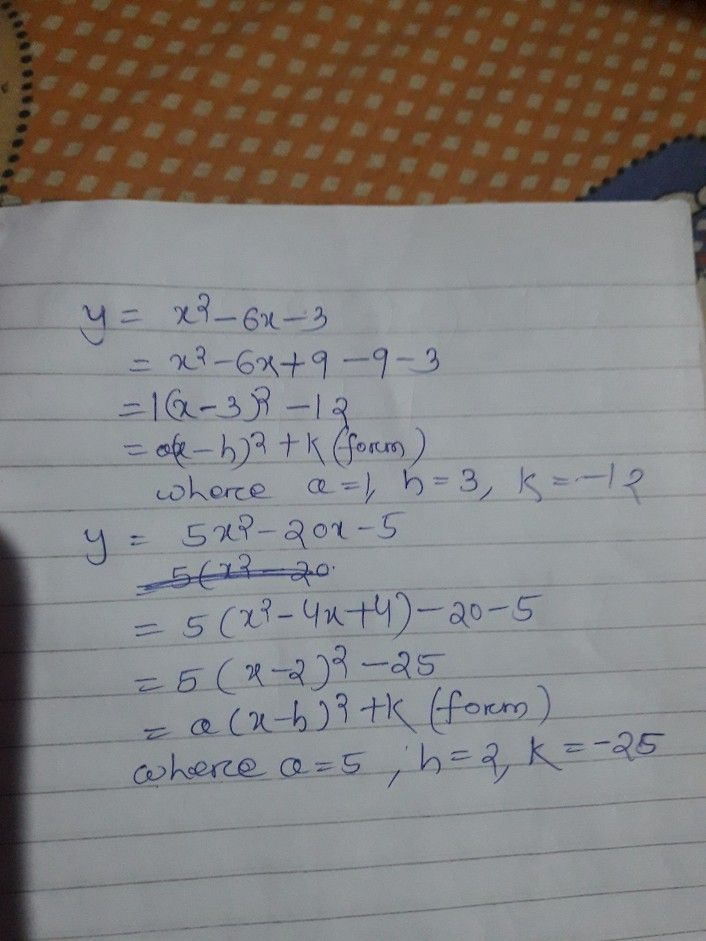Symbol
Problem$3$ Learning Task $3$ Transform the quadratic function defined by $y=ax^{2+bx+}$ c into the form $y=a\left(x-h\right)^{2+k}$ $1.$ $y=x^{2}-6x-3$ $2$ $y=5x2$ $-20x-5$
7th-9th grade
Algebra
Search count: 107
Question content
Y=5x2-20x-5
SolutionQanda teacher - kamalsahooStudent
Thank u sir